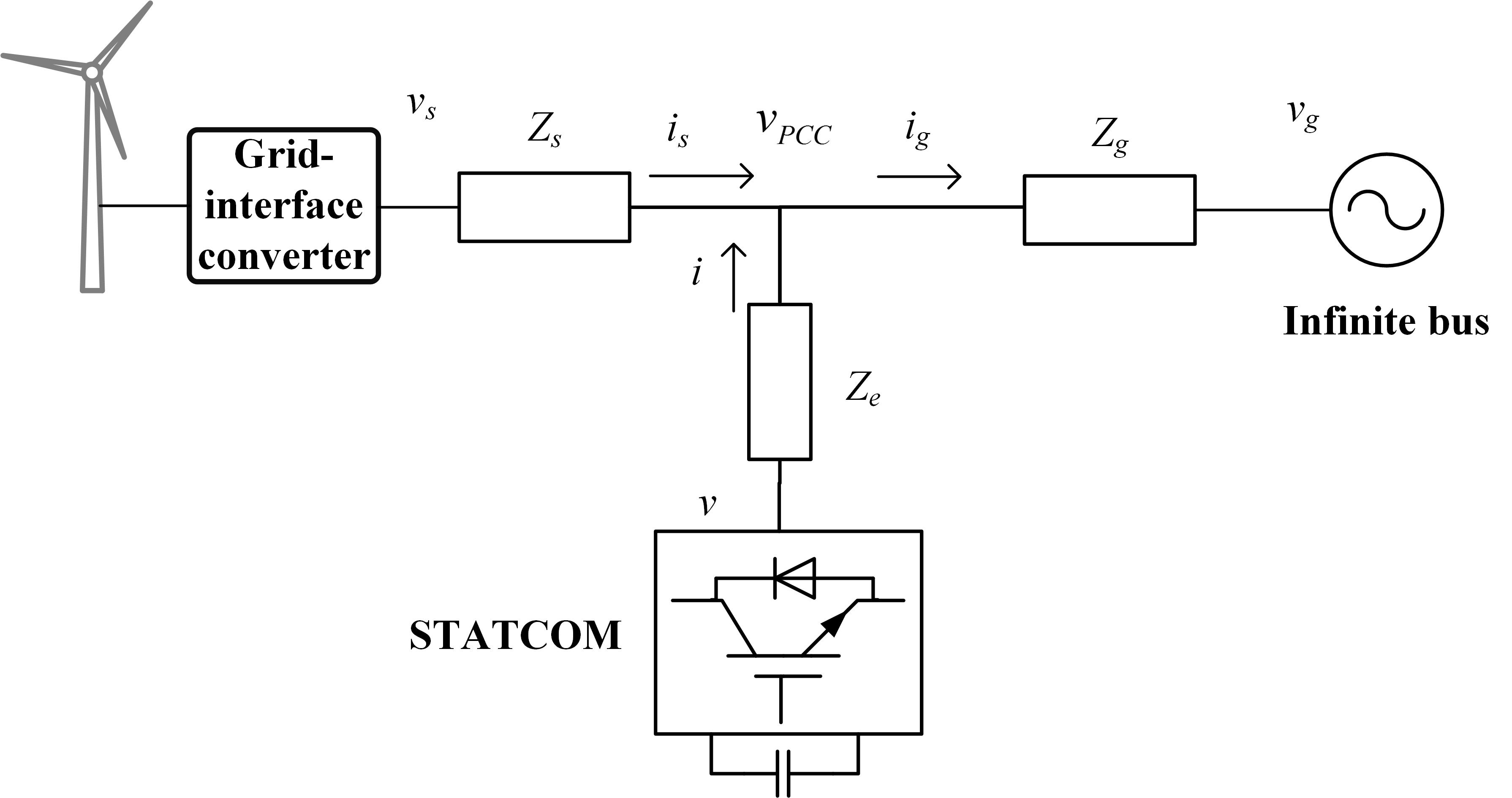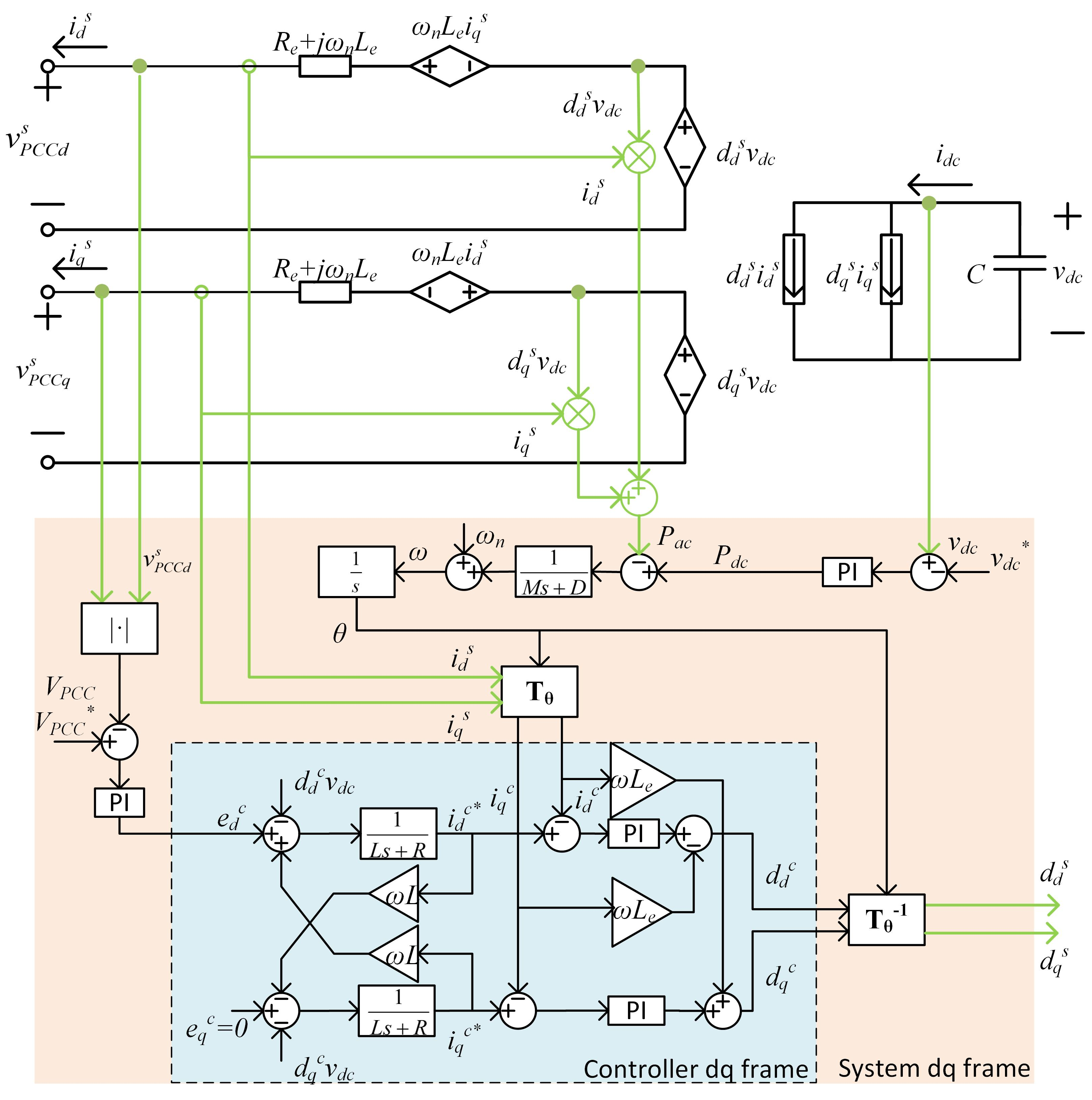Contact Us
LIBRARY

# Analysis and Design of a Virtual Synchronous Machine Based STATCOM Control-lerFig. 1 Single Line Diagram of the Test Bed
This paper extends the virtual synchronous machine (VSM) concept, recently proposed as an alternative means to synchronize grid-connected inverters, by developing a VSM-based STAT-COM controller operating as synchronous condenser. In this article, the test bed studied is as shown in Fig. 1, where the wind farm is providing power to an infinite bus bar with a shunt-connected STATCOM tracking and regulating the PCC voltage vPCC. To this end, a mathematical model is derived and used to analyze the inherent dynamics of the VSM-based STATCOM controller. The dynamics are then used to formulate design guidelines that further detach the proposed method from the perceived physical constraints introduced by the VSM concept. In order to gain a better understanding, we can transform the test bed and the proposed controller in the abc frame into the d-q frame, as shown in Fig. 2. It should be noted that there are two d-q frames: the system d-q frame with superscript s rotating at the nominal frequency ωn, and the controller d-q frame with superscript c at frequency ω, and the angular difference , which is necessary for the coordinate transformation T between both d-q frames. Based on the model in the d-q frame, the effects of each parameter are studied by investigating the Bode plots of the control loops, raising some discussion about trade-offs in parameter selections.Fig. 2 Power Stage with VSM Controller in d-q FrameINDUSTRY PARTNERS